### Engineering Graphics - Projections of a Straight Line

Projections of a Straight Line

Introduction

The shortest distance between any two points is called a "straight line". Different surfaces and planes form the configuration or shape of any object. Revolving or moving straight lines in different ways obtains these surfaces and planes. Thus a straight line is the basic conceptual

figure using which any object like a machine component or a structural element is represented.

Thus projection of a straight line is the foundation of Engineering Drawing. projections of two points gives the projection of the straight line joining the two points.

As per ISO convention the first angle of projection is used.

Objectives

To Define straight line.

To Draw the projections of a straight line located at different positions with respect to the VP and the HP.

Perpendicular to the HP and Parallel to the VP

A Line AB 25mm is parallel to the VP and perpendicular to the HP. Point A is 35mm above the HP and 20mm in front of the VP. B is 10 mm above the HP. Draw its projections.

1. Draw the line XY.

2. Draw a line perpendicular to XY using a 2H pencil.

3. Mark b' 10mm above XY on the perpendicular line.

4. Mark a' 25mm above b'.

5. a' b' is the front view, join a', b' using a H pencil.

6. Mark a (b) 20mm below XY; a (b) is the top View.

7. Erase the unwanted Lines.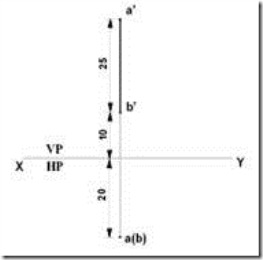Perpendicular to the VP and Parallel to the HP

A Line AB of length 25mm is perpendicular to the VP and Parallel to the HP. The point A is 20mm above the HP and 10mm in front of the VP. Draw its projections.

1. Draw the line XY.

2. Draw a line perpendicular to XY using a 2H pencil.

3. Mark "a" 10mm below XY on the perpendicular line.

4. Mark "b" 25mm below "a".

5. Join "a" and "b" using an H pencil to get the top view.

6. Mark a' (b') 20mm above XY line on the perpendicular line

7. Erase the unwanted Lines.Parallel to the HP and the VP

A Line CD 30mm long is parallel to both planes. The line is 40mm above the HP and 25mm in front of the VP. Draw its projections.

1. Draw the line XY.

2. Draw a line perpendicular to XY using a 2H pencil.

3. Draw another perpendicular line 30mm from the previous line.

4. Mark “c'” and “d' ” on the Perpendicular lines and join them to get the front view.

5. Mark “c” 25mm below line XY; join “c” and “d” to get the top view.

6. Erase the unwanted Lines.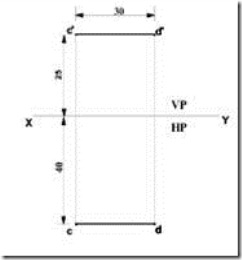Parallel to the HP and Inclined to the VP

A line PQ of length 40mm is parallel to the HP and inclined at an angle of 35° to the VP. The end P is 20mm above the HP and 15mm in front of the VP. Draw its projections.

1. Draw the line XY.

2. Draw a line perpendicular to XY using a 2H pencil

3. Mark “p'” and “p” respectively 15 mm above XY and 20mm below XY on the perpendicular line.

4. From “p” draw a line at an angle of 35° to XY and mark

“q” such that pq = 40mm = true length.

5. pq is the top view of the given line in the I-Quadrant.

6. From “q” draw a projector (perpendicular line) to intersect the horizontal line drawn from “p'” at “q'”.

7. p' q ' is the front view.

8. Erase the unwanted line.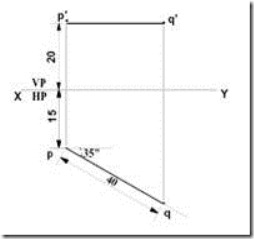Parallel to the VP and Inclined to the HP

A line PQ of length 40mm is parallel to the VP and inclined at an angle of 30° to the HP. The end P is 15mm above the HP and 20mm in front of the VP. Draw its projections.

1. Draw the line XY.

2. Draw a perpendicular line to XY using 2H pencil.

3. Mark p' & p 15mm above XY & 20mm below XY on the perpendicular line.

4. From p' draw a line at angle of 30° to XY and mark q'. such that p'q'= 40mm = True length

5. p' q' is the required Front View

6. From q' draw a projector (perpendicular line) to intersect the horizontal line drawn from p at q.

7. pq is the required Top View

8. Erase the unwanted Line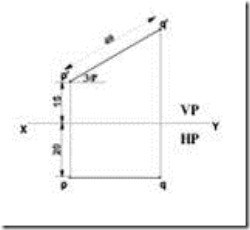Inclined to the HP and the VP

A line PQ of length 40mm is inclined to both the VP and the HP. The Line is inclined at 300 to HP and 450 to VP. The Point P is 20mm above HP and 30mm in front of VP. Draw its projections.

1. Draw the line XY.

2. Mark “p” below XY line and draw 45° line and mark q2 at

80mm

3. Mark “p'” above XY line and draw 30° line and mark “q1' ”

at 80mm

4. Draw locus of “q1' ” and “q2”

5. Project from “q1' ” and “p” as centre rotate, it cuts locus of “q2” at “q”

6. Joint “p” and “q” to get top view

7. Project from “q2” and “p' ” as centre rotate, it cuts locus of “q1' ” at “q' ”

8. Joint “p’” and “q’” to get front view.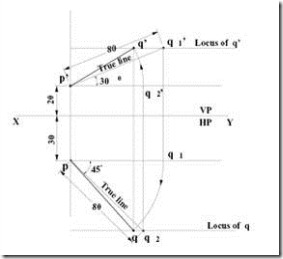#### 1 comment:

1.